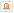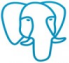설문조사
PostgreSQL/PPAS 관련 듣고 싶은 교육은
 SQL PL/PGSQL Administration(for DBA) Backup & Recovery Performance Tuning Oracle Migration및 비교 Replication 기타(듣고 싶은 강좌게시판에)

 총 게시물 149건, 최근 0 건# Speeding Up Updates with CTE

글쓴이 :

This is a case study on improving the performance of an UPDATE query.

The original query performed well in ORACLE. In Oracle the elapsed time was about 0.2 seconds. After migrating to PostgreSQL, it took 4.8 seconds to get the results. Below are the sample tables and data.

We start with a table to update, a view, and two tables to make up the view:

drop table if exists t1 cascade;

drop table if exists t2 cascade;

create table t1

as

select trunc((i-1)/15) n1,

trunc((i-1)/15) n2,

from generate_series(1,30000) a(i);

create table t2

as

select

mod(i,200)         n1,

mod(i,200)         n2,

from generate_series(1,30000) a(i);

create index t1_i1 on t1(n1);

create index t2_i1 on t2(n1);

create or replace view v1

as

select distinct

t1.n1 t1n1, t1.n2 t1n2, t2.n2 t2n2

from

t1, t2

where

t1.n1 = t2.n1

;

drop table if exists t3 ;

create table  t3

as

select * from v1

;

analyze  t1;

analyze  t2;

analyze  t3;

Below is the query we have to investigate followed by its execution plan.

The plan was gained by explain(analyze, buffers, costs off).

update t3

set t2n2 = (

select  v1.t2n2

from    v1

where   v1.t1n1 = t3.t1n1

and     v1.t1n2 = t3.t1n2

)

;

Here is the execution plan

Update on t3 (actual time=4797.836..4797.838 rows=0 loops=1)

Buffers: shared hit=2433875 dirtied=5

->  Seq Scan on t3 (actual time=30.179..4795.885 rows=200 loops=1)

Buffers: shared hit=2433671

SubPlan 1

->  Subquery Scan on v1 (actual time=23.786..23.976 rows=1 loops=200)

Buffers: shared hit=2433667

->  Unique (actual time=23.785..23.975 rows=1 loops=200)

Buffers: shared hit=2433667

->  Sort (actual time=23.765..23.842 rows=2250 loops=200)

Sort Key: t2.n2

Sort Method: quicksort  Memory: 272kB

Buffers: shared hit=2433667

->  Nested Loop (actual time=0.017..23.464 rows=2250 loops=200)

Buffers: shared hit=2433664

->  Index Scan using t1_i1 on t1 (al time=0.008..0.021 rows=15 loops=200

Index Cond: (n1 = t3.t1n1)

Filter: (n2 = t3.t1n2)

Buffers: shared hit=664

->  Seq Scan on t2 (actual time=0.005..1.549 rows=150 loops=3000)

Filter: ((n1)::double precision = t3.t1n1)

Rows Removed by Filter: 29850

Buffers: shared hit=2433000

Planning:

Buffers: shared hit=221 dirtied=1

Planning Time: 2.031 ms

Execution Time: 4798.103 ms

There are some points to note from this execution plan:

- the Subquery Scan on v1 has looped 200 times (there are 200 rows in table t3, the subquery runs once per row)

- the elapsed time per SubPlan1 is 23.976 ms, the elapsed time of the subquery in total can be calculated like this: 23.976 ms X 200 = 4795.2 ms

- the predicate t3.t1n1 has been pushed inside the view v1, so the optimizer has decided to use the index t1_i1 in accessing t1 table.

- after accessing t1_i1 and filtering with the n2=t3.t1n2 predicate, the optimizer got 15 rows.

- and then it accessed the t2 table

The problem in the execution plan above is that we have to loop the subquery 200 times, and the elapsed time 23.976 ms is not small considering the number of loops. Consequently, the block I/O in the subquery is unreasonably big. (I know that you may wonder how many block I/O numbers are big. I personally investigate the execution plan when the block I/O is above 10,000. When the block I/O is under 10000, I don't care whether it has an efficient execution plan.)

Now let's see what the SQL and the plan look like if we want PostgreSQL to create the entire v1 result set and use that to update the t3 table.

update t3

set t2n2 = (

select  v1.t2n2

from     (select distinct

t1.n1 t1n1, t1.n2 t1n2, t2.n2 t2n2

from

t1, t2

where

t1.n1 = t2.n1

offset 0     --You have to put this to prevent subquery collapse

) v1

where   v1.t1n1 = t3.t1n1

and     v1.t1n2 = t3.t1n2

);

Update on t3 (actual time=122.561..122.567 rows=0 loops=1)

Buffers: shared hit=1877 dirtied=5

->  Seq Scan on t3 (actual time=106.784..122.266 rows=200 loops=1)

Buffers: shared hit=1673

SubPlan 1

->  Subquery Scan on v1 (actual time=0.572..0.611 rows=1 loops=200)

Filter: ((v1.t1n1 = t3.t1n1) AND (v1.t1n2 = t3.t1n2))

Rows Removed by Filter: 199

Buffers: shared hit=1669

->  HashAggregate (actual time=0.533..0.602 rows=200 loops=200)

Group Key: t1.n1, t1.n2, t2.n2

Batches: 1  Memory Usage: 801kB

Buffers: shared hit=1669

->  Hash Join (actual time=9.115..51.160 rows=450000 loops=1)

Hash Cond: ((t2.n1)::double precision = t1.n1)

Buffers: shared hit=1669

->  Seq Scan on t2 (actual time=0.003..1.949 rows=30000 loops=1)

Buffers: shared hit=811

->  Hash (actual time=9.007..9.008 rows=30000 loops=1)

Buckets: 32768  Batches: 1  Memory Usage: 1663kB

Buffers: shared hit=858

->  Seq Scan on t1 (actual time=0.005..5.782 rows=30000 loops=1)

Buffers: shared hit=858

Planning:

Buffers: shared hit=12

Planning Time: 0.179 ms

Execution Time: 123.600 ms

The most important figure to note in this execution plan is that 1673 shared buffer visits - clearly we have done something very efficient. As we intended, the predicates t3.t1n1 and t3.t1n2 have not been pushed inside the view v1. So in order to create the entire v1 result set, the optimizer joined the two tables with Hash Join method. So it took 0.611 ms per Subquery Scan on v1. The elapsed time of the subquery in total can be calculated like this: 0.611 ms X 200 = 122.2 ms

One of the ways to create the entire v1 result set is to use a Common Table Expression. Sometimes a CTE is faster than a Subquery.

Below is the SQL followed by its execution plan.

update t3

set t2n2 = (

with v0 as materialized (--without materialized the subquery collapses

select

t1n1, t1n2, t2n2

from v1

)

select

t2n2

from

v0

where   v0.t1n1 = t3.t1n1

and     v0.t1n2 = t3.t1n2

);

Update on t3 (actual time=108.168..108.171 rows=0 loops=1)

Buffers: shared hit=1877 dirtied=5

->  Seq Scan on t3 (actual time=105.968..107.995 rows=200 loops=1)

Buffers: shared hit=1673

SubPlan 2

->  CTE Scan on v0 (actual time=0.535..0.540 rows=1 loops=200)

Filter: ((t1n1 = t3.t1n1) AND (t1n2 = t3.t1n2))

Rows Removed by Filter: 199

Buffers: shared hit=1669

CTE v0

->  HashAggregate (actual time=105.822..105.910 rows=200 loops=1)

Group Key: t1.n1, t1.n2, t2.n2

Batches: 1  Memory Usage: 801kB

Buffers: shared hit=1669

->  Hash Join (actual time=6.984..49.351 rows=450000 loops=1)

Hash Cond: ((t2.n1)::double precision = t1.n1)

Buffers: shared hit=1669

->  Seq Scan on t2 (actual time=0.002..1.902 rows=30000 loops=1)

Buffers: shared hit=811

->  Hash (actual time=6.956..6.957 rows=30000 loops=1)

Buckets: 32768  Batches: 1  Memory Usage: 1663kB

Buffers: shared hit=858

->  Seq Scan on t1 (actual time=0.002..3.802 rows=30000 loops=1)

Buffers: shared hit=858

Planning:

Buffers: shared hit=6 dirtied=1

Planning Time: 0.193 ms

Execution Time: 108.423 ms

It has almost the same execution plan. The shared buffer hit number 1673 is the same. But the elapsed time improved a little bit from 123.6 ms to 108.4 ms. I don't know why the query using a CTE is faster. I would appreciate it if someone explains the reason. My estimation is that the result set of the subquery stays in the work_mem area because it hash joined, on the other hand the result set of the CTE stays in the shared_buffer but I am not sure.

FOOTNOTE

A day after posting this note, I realized that the problematic 1st query was not the standard SQL. The SQL is just the Oracle dialect which can be processed only in ORACLE DB system. PostgreSQL happens to be able to interpret the Oracle dialect. So I changed the problematic SQL into the standard SQL that ANSI officially adopted.

Below is the standard version of the problematic SQL.

update t3

set t2n2 = v1.t2n2

from v1

where v1.t1n1 = t3.t1n1

and  v1.t1n2 = t3.t1n2;

Below is the execution plan.

Update on t3 (actual time=105.336..105.338 rows=0 loops=1)

Buffers: shared hit=1926

->  Hash Join (actual time=104.867..105.033 rows=200 loops=1)

Hash Cond: ((v1.t1n1 = t3.t1n1) AND (v1.t1n2 = t3.t1n2))

Buffers: shared hit=1673

->  Subquery Scan on v1 (actual time=104.782..104.903 rows=200 loops=1)

Buffers: shared hit=1669

->  HashAggregate (actual time=104.776..104.868 rows=200 loops=1)

Group Key: t1.n1, t1.n2, t2.n2

Batches: 1  Memory Usage: 801kB

Buffers: shared hit=1669

->  Hash Join (actual time=7.389..48.973 rows=450000 loops=1)

Hash Cond: ((t2.n1)::double precision = t1.n1)

Buffers: shared hit=1669

->  Seq Scan on t2 (actual time=0.002..1.802 rows=30000 loops=1)

Buffers: shared hit=811

->  Hash (actual time=7.367..7.367 rows=30000 loops=1)

Buckets: 32768  Batches: 1  Memory Usage: 1663kB

Buffers: shared hit=858

->  Seq Scan on t1 (actual time=0.002..3.740 rows=30000 loops=1)

Buffers: shared hit=858

->  Hash (actual time=0.080..0.080 rows=200 loops=1)

Buckets: 1024  Batches: 1  Memory Usage: 19kB

Buffers: shared hit=4

->  Seq Scan on t3 (actual time=0.011..0.051 rows=200 loops=1)

Buffers: shared hit=4

Planning Time: 0.138 ms

Execution Time: 105.503 ms모델광2021-05-20 (목) 23:07
 Wow! Just by using the standard SQL, we could give the optimizer better information it needs to make an efficient plan. Actually the fuss I made in this note was a waste of time. You could improve the performance of the problematic SQL just by rewriting it in the standard format. Anyway while writing this note, I got a lesson. When you are in a project where you change the DBMS, try to follow the SQL standard. If you use the ORACLE dialect, the optimizer may produce an inefficient execution plan.
댓글주소PostgresDBA2021-05-21 (금) 13:50
 직접 테스트해보시면서 영어로 작성하시는건가? 능력자시네요. 부럽습니다
댓글주소모델광2021-05-22 (토) 10:31
 다른 사람들이 보는 게시판에 저의 생각을 올려 놓으니, 저의 생각에도 많은 오류가 있었음을 깨닫게 되네요. 다른 사람들이 볼거라고 생각하니 다 쓰고 나서도 내가 적어 놓은게 맞나 하고 다시 생각하게 됩니다. 아마 고수들이 보면 틀리거나 더 개선할 수 있는 내용도 많을 것입니다. 위 내용만 보더라도 표준 SQL 쓰면 간단히 해결할 수 있던것을 실제 프로젝트에서는 SQL을 Oracle style로 적용했었습니다. 저도 설명할 수 없는 부분은 외국의 커뮤니티 사이트에 물어볼때 활용하기 위해서 영어로 작성하고 있습니다.
댓글주소